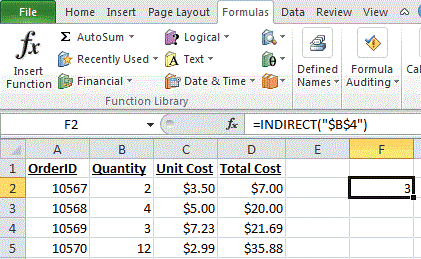## Excel Office

Excel How Tos, Tutorials, Tips & Tricks, Shortcuts

# How to use Excel INDIRECT Function

This Excel tutorial explains how to use the INDIRECT function with syntax and examples.

## Excel INDIRECT function Description

Microsoft Excel INDIRECT function returns the reference to a cell based on its string representation.

The INDIRECT function is a built-in function in Excel that is categorized as a Lookup/Reference Function that can be entered as part of a formula in a cell of a worksheet.

Worked Example:   How to translate letters to numbers in ExcelExplanation: Based on the example above, the INDIRECT function would return:

```=INDIRECT("\$B\$4")
Result: 3

=INDIRECT("A5")
Result: 10570

=INDIRECT("A5", TRUE)
Result: 10570

=INDIRECT("R2C3", FALSE)
Result: \$3.50```

### Syntax

The syntax for the INDIRECT function in Microsoft Excel is:

`INDIRECT( string_reference, [ref_style] )`

### Arguments

string_reference
A textual representation of a cell reference.
ref_style
Optional. It is either a TRUE or FALSE value. TRUE indicates that string_reference will be interpreted as an A1-style reference. FALSE indicates that string_reference will be interpreted as an R1C1-style reference. If this parameter is omitted, it will interpret string_reference as an A1-style.
Worked Example:   Example of COUNTIFS with variable table column in Excel